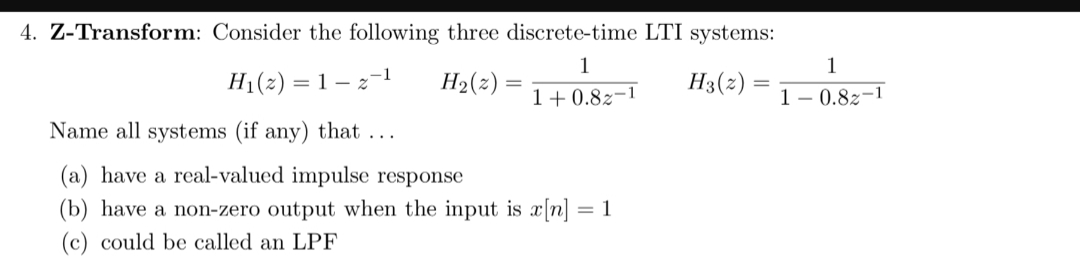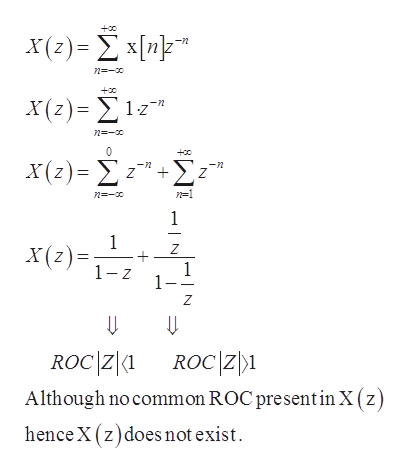# 4. Z-Transform: Consider the following three discrete-time LTI systems:11H1(2) 1H2(2)H3(2)=1 0.82-11 - 0.82-1Name all systems (if any) that ...(a) have a real-valued impulse response(b) have a non-zero output when the input is x[n]= 1(c) could be called an LPF

Question
54 viewshelp_outlineImage Transcriptionclose4. Z-Transform: Consider the following three discrete-time LTI systems: 1 1 H1(2) 1 H2(2) H3(2) = 1 0.82-1 1 - 0.82-1 Name all systems (if any) that ... (a) have a real-valued impulse response (b) have a non-zero output when the input is x[n] = 1 (c) could be called an LPF fullscreen
check_circle

Step 1

Given discrete-time LTI systems are

Step 2

Part (a)-

although

filter co-efficient of H1(z) is -1

filter co-efficient of H2(z) is +0.8

filter co-efficient of H3(z) is -0.8

so, all three discrete-time LTI system have real valued filter co-efficient therefore all have real valued impulse response.

Step 3

Part (b)-

Given Input is, x[n]=1

z-t...help_outlineImage Transcriptionclose+o X(?) - Σ x[up" X(2) - Σ1z"η X(2)-Σ -n n1 n- 1 1 X(z) 1 z Z Z ROC Z1 Although no common ROC present inX (z) ROCZ1 hence X (z)does not exist N fullscreen

### Want to see the full answer?

See Solution

#### Want to see this answer and more?

Solutions are written by subject experts who are available 24/7. Questions are typically answered within 1 hour.*

See Solution
*Response times may vary by subject and question.
Tagged in

### Electrical Engineering